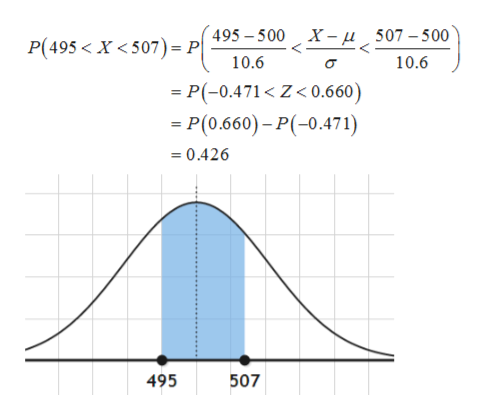# In a recent year, the MCAT total scores were normally distributed, with a mean of 500 and a standard deviation of 10.6.  Find the probability that a randomly selected medical student who took the MCAT has a total score that is:a.  less than 489b.  between 495 and 507c.  more than 522

Question
193 views

In a recent year, the MCAT total scores were normally distributed, with a mean of 500 and a standard deviation of 10.6.  Find the probability that a randomly selected medical student who took the MCAT has a total score that is:

a.  less than 489

b.  between 495 and 507

c.  more than 522

check_circle

Step 1

Consider,

Let X be a random variable that represents the total score which is normally distributed that is,

Step 2

(a)

The probability that randomly selected students have score less than 489:

Step 3

(b)

The probability that randomly selected stud...help_outlineImage Transcriptionclose495-500 X-u 507-500 P(495 < X <507)= P| 10.6 10.6 - P(-0.471< Z< 0.660) -P(0.660)-P(-0.471) 0.426 495 507 fullscreen

### Want to see the full answer?

See Solution

#### Want to see this answer and more?

Solutions are written by subject experts who are available 24/7. Questions are typically answered within 1 hour.*

See Solution
*Response times may vary by subject and question.
Tagged in

### Statistics